# AV1(DAV1D) 解码详解(三)之CDEF

CDEF(constrained directional enhancement)约束方向增强滤波器是 AV1 里的几种滤波器之一， SPEC 中定义的该部分在 7.15 CDEF process 中。AOM 代码中在 cdef.c 相关的文件中。除此外，还可以参考 The AV1 CONSTRAINED DIRECTIONAL ENHANCEMENT FILTER论文，本文就是基于上面三个部分分析的结果。

## CDEF 介绍

CDEF 的主要目的是过滤掉编码杂音，同时保留图像的详细内容。AV1 中采用的方法是非线性空间滤波器，该滤波器的设计非常适用于矢量化，即可以使用 SIMD 操作，而其他非线性滤波器（如中值滤波器和双边滤波器）并非如此。

CDEF 滤波器的设计基于以下观察：编码图像中杂音的数量通常与量化步长大致成比例，图像详细内容的数量是输入图像的一个属性，保留在量化过的图像中的最小的实际信息量也与量化成一定的比例。对于给定的步长，杂音的幅度要比细节的幅度小很多。

CDEF 首先判断每个块的方向，之后沿着判断的方向自适应的滤波，并沿着判断的方向小幅度旋转 45 度，滤波强度明确表示，对模糊的高度控制。

CDEF 是基于之前提到的两个环路滤波器，结合的滤波器用在了 AV1 Codec 中。

• CDEF Block 处理
• CDEF Direction 处理
• CDEF Filter 处理

## 方向查找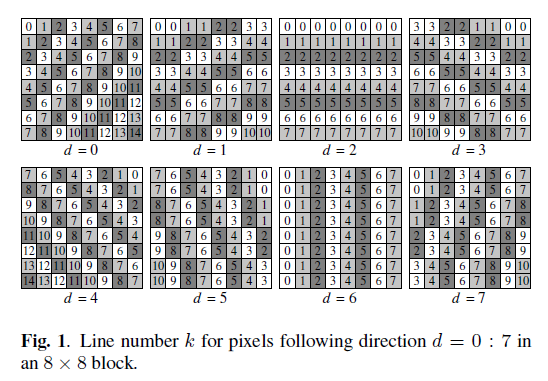• $x_p$ 是像素$p$的值
• $P_{d,k}$是在方向$d$上第$k$行的一组像素值
• $N_{d,k}$是相对$P_{d,k}$对应的基数，如$N_{1,0}=2, N_{1,4}=8$

SSD的计算公式如下：$E_{d}^2 = \sum_{k} \big[\sum_{p \in P_{d,k}} \big( x_{p} - u_{d,k}\big)^2 \big]$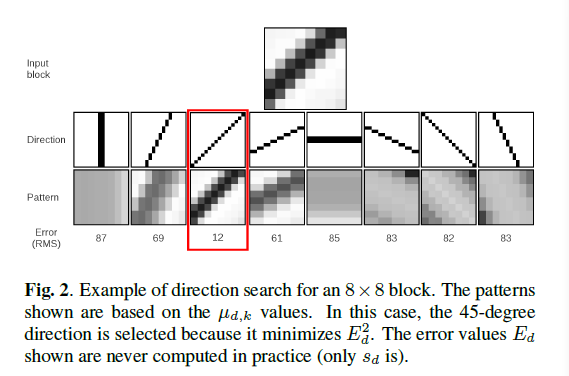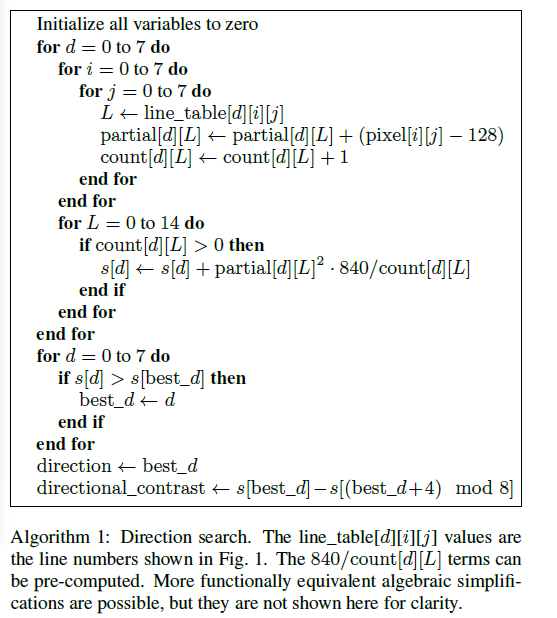## 非线性低通滤波器

CDEF 使用非线性低通滤波器，去除编码杂音的同时不会模糊块的边缘。AV1 根据特定方向寻找滤波器抽头位置，同时当滤波器运用到块边界时，要防止过度模糊。使用非线性低通滤波器，在滤波像素偏差过大时，就不再对该像素过度强调。

### 定向滤波器

primary taps 沿着方向 d，它的系数如上面图 4 所示。对 primary taps，对不同的 strength，会有不同的系数，对于1/3/5的strength，与2/4/6的strength，系数是不同的。secondary tpas 会形成一个十字架，是方向 d 旋转 45° 后得到，系数如图 5。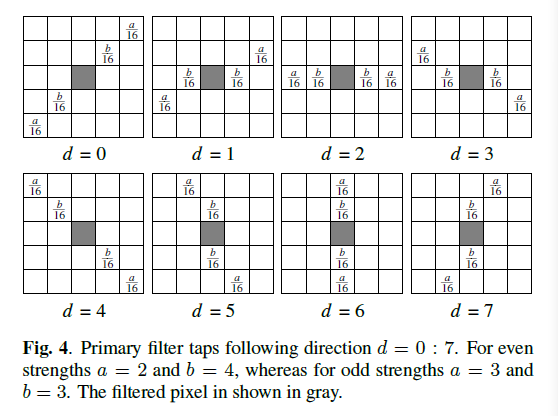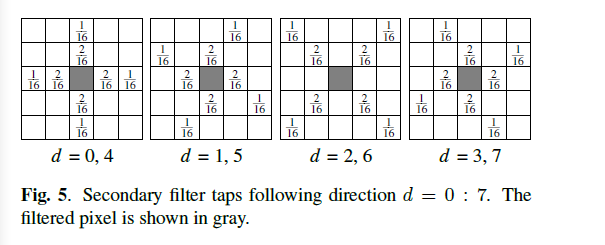2-D CDEF 滤波器公式如下：

$y(i,j) = x(i,j) + round( \sum_{m,n} w_{d,m,n}^{(p)} f(x(m,n) -x(i, j), S^{(p)}, D) + \sum_{m,n} w_{d,m,n}^{(s)} f(x(m,n) -x(i,j), S^{(s)}, D))$

• $S_{p}$和$S_{s}$是 primary 和 secondary 抽头的 strength。

### 代码实现分析

#### 方向查找实现

DAV1D 工程里，对 CDEF 方向查找，完成了 C 代码实现和对 8bit 码流的汇编优化，对10bit优化并没有完成。